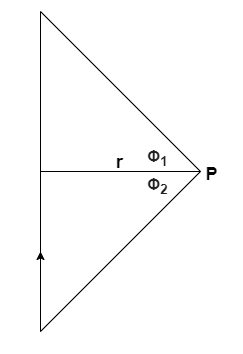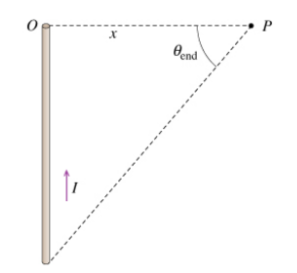# Problem: A steady current I is flowing through a straight wire of finite length. Now find B2, the magnetic field generated by this wire at a point P located a distance x from either end of the wire. Assume that at P the angle subtended from the end of the wire to the other end is θend as shown in the diagram.

###### FREE Expert Solution

The magnetic field at point P due to a finite wire carrying current:In this problem:

Φ1 = 0

Φ2 = θend

99% (48 ratings)###### Problem Details

A steady current I is flowing through a straight wire of finite length.Now find B2, the magnetic field generated by this wire at a point P located a distance x from either end of the wire. Assume that at P the angle subtended from the end of the wire to the other end is θend as shown in the diagram.